Program for all operations on circular linked list in C

• Difficulty Level : Easy
• Last Updated : 18 Aug, 2021

In a Circular linked list, every element has a link to its next element in the sequence and the last element has a link to the first element. A circular linked list is similar to the singly linked list except that the last node points to the first node. Below is the image to illustrate the same: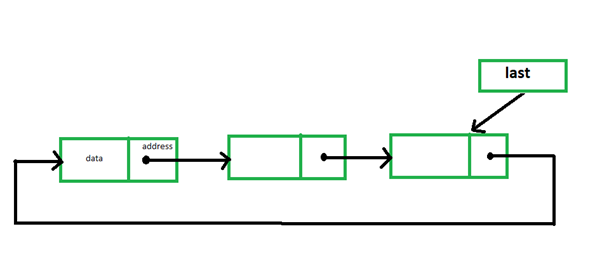Some common operations of a circular linked list are implemented below:

Insertion at the beginning: Inserting a new node as the first node. The next pointer of last will point to this node and this new node will point to the previous first node.

C

 // C program for the above operation#include #include  // Structure of a linked list nodestruct node {    int info;    struct node* next;}; // Pointer to last node in the liststruct node* last = NULL; // Function to insert a node in the// starting of the listvoid insertAtFront(){    // Stores the number to be inserted    int data;     // Initialize a new node    struct node* temp;    temp = (struct node*)malloc(sizeof(struct node));     // Input data    printf("\nEnter data to be "           "inserted: \n");    scanf("%d", &data);     // If the new node is the only    // node in the list    if (last == NULL) {        temp->info = data;        temp->next = temp;        last = temp;    }     // Else last node contains the    // reference of the new node and    // new node contains the reference    // of the previous first node    else {        temp->info = data;        temp->next = last->next;         // last node now has reference        // of the new node temp        last->next = temp;    }} // Function to print the listvoid viewList(){    // If list is empty    if (last == NULL)        printf("\nList is empty\n");     // Else print the list    else {        struct node* temp;        temp = last->next;         // While first node is not        // reached again, print,        // since the list is circular        do {            printf("\nData = %d", temp->info);            temp = temp->next;        } while (temp != last->next);    }} // Driver Codeint main(){    // Function Call    insertAtFront();    insertAtFront();    insertAtFront();     // Print list    viewList();     return 0;}

Output: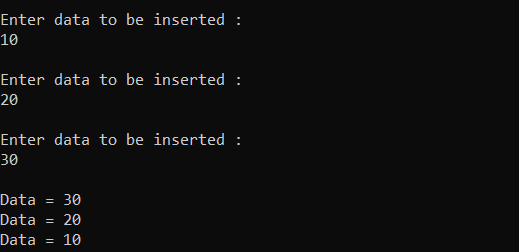Insertion at the end: Inserting a new node as the last node. The next pointer of last will point to this node and this new node will point to the first node.

C

 // C program for the above operation#include #include  // Structure of a linked list nodestruct node {    int info;    struct node* next;}; // Pointer to last node in the liststruct node* last = NULL; // Function to add a new node at the// end of the listvoid addatlast(){    // Stores number to be inserted    int data;     // Initialize a new node    struct node* temp;    temp = (struct node*)malloc(sizeof(struct node));     // Input data    printf("\nEnter data to be "           "inserted : \n");    scanf("%d", &data);     // If the new node is the    // only node in the list    if (last == NULL) {        temp->info = data;        temp->next = temp;        last = temp;    }     // Else the new node will be the    // last node and will contain    // the reference of head node    else {        temp->info = data;        temp->next = last->next;        last->next = temp;        last = temp;    }} // Function to print the listvoid viewList(){    // If list is empty    if (last == NULL)        printf("\nList is empty\n");     // Else print the list    else {        struct node* temp;        temp = last->next;        do {            printf("\nData = %d",                   temp->info);            temp = temp->next;        } while (temp != last->next);    }} // Driver Codeint main(){    // Function Call    addatlast();    addatlast();    addatlast();     // Print list    viewList();     return 0;}

Output: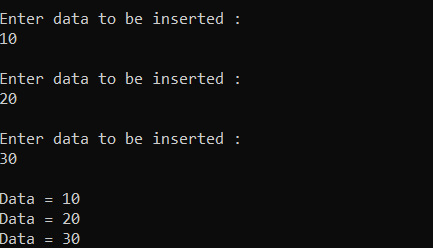Insertion after a specific element: Below is the program to insert a node after a specified node in the linked list.

C

 // C program for the above operation#include #include  // Structure of a linked list nodestruct node {    int info;    struct node* next;}; // Pointer to last node in liststruct node* last = NULL; // Function to add a new node// at the end of the listvoid addatlast(){    // Stores number to be inserted    int data;     // Initialize a new node    struct node* temp;    temp = (struct node*)malloc(sizeof(struct node));     // Input data    printf("\nEnter data to be inserted : \n");    scanf("%d", &data);     // If the new node is the    // only node in the list    if (last == NULL) {        temp->info = data;        temp->next = temp;        last = temp;    }     // Else the new node will be the    // last node and will contain    // the reference of head node    else {        temp->info = data;        temp->next = last->next;        last->next = temp;        last = temp;    }} // Function to insert after any// specified elementvoid insertafter(){    // Stores data and element after    // which new node is to be inserted    int data, value;     // Initialize a new node    struct node *temp, *n;     // Input data    printf("\nEnter number after which"           " you want to enter number: \n");    scanf("%d", &value);    temp = last->next;     do {         // Element after which node is        // to be inserted is found        if (temp->info == value) {            n = (struct node*)malloc(sizeof(struct node));             // Input Data            printf("\nEnter data to be"                   " inserted : \n");            scanf("%d", &data);            n->info = data;            n->next = temp->next;            temp->next = n;             // If temp is the last node            // so now n will become the            // last node            if (temp == last)                last = n;            break;        }        else            temp = temp->next;    } while (temp != last->next);} // Function to print the listvoid viewList(){    // If list is empty    if (last == NULL)        printf("\nList is empty\n");     // Else print the list    else {        struct node* temp;        temp = last->next;        do {            printf("\nData = %d",                   temp->info);            temp = temp->next;        } while (temp != last->next);    }} // Driver Codeint main(){    // Initialize the list    addatlast();    addatlast();    addatlast();     // Function Call    insertafter();     // Print list    viewList();     return 0;}

Output: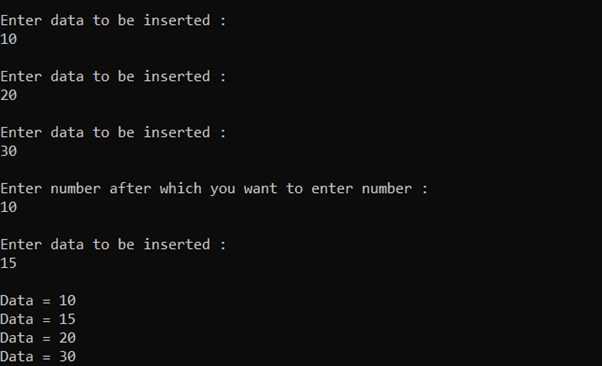Delete first element: Deleting the first node of the linked list. For this, the next pointer of last will point to the second node of the linked list.  Below is the program for the same:

C

 // C program for the above operation#include #include  // Structure of a linked list nodestruct node {    int info;    struct node* next;}; // Pointer to last node in liststruct node* last = NULL; // Function to add a new node// at the end of the listvoid addatlast(){    // Stores number to be inserted    int data;     // Initialize a new node    struct node* temp;    temp = (struct node*)malloc(sizeof(struct node));     // Input data    printf("\nEnter data to be"           " inserted: \n");    scanf("%d", &data);     // If the new node is the only    // node in the list    if (last == NULL) {        temp->info = data;        temp->next = temp;        last = temp;    }     // Else the new node will be the    // last node and will contain    // the reference of head node    else {        temp->info = data;        temp->next = last->next;        last->next = temp;        last = temp;    }} // Function to delete the first// element of the listvoid deletefirst(){    struct node* temp;     // If list is empty    if (last == NULL)        printf("\nList is empty.\n");     // Else last node now contains    // reference of the second node    // in the list because the    // list is circular    else {        temp = last->next;        last->next = temp->next;        free(temp);    }} // Function to print the listvoid viewList(){    // If list is empty    if (last == NULL)        printf("\nList is empty\n");     // Else print the list    else {        struct node* temp;        temp = last->next;        do {            printf("\nData = %d",                   temp->info);            temp = temp->next;        } while (temp != last->next);    }} // Driver Codeint main(){    // Initialize the list    addatlast();    addatlast();    addatlast();     // Function Call    deletefirst();     // Print list    viewList();     return 0;}

Output: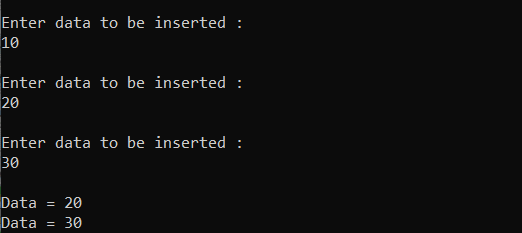Delete the last element: Deleting the last node of the linked list. For this, the second last node will point to the first node of the list. Below is the program for the same:

C

 // C program for the above operation#include #include  // Structure of a linked list nodestruct node {    int info;    struct node* next;}; // Pointer to last node in liststruct node* last = NULL; // Function to add a new node// at the end of the listvoid addatlast(){    // Stores number to be inserted    int data;     // Initialize a new node    struct node* temp;    temp = (struct node*)malloc(sizeof(struct node));     // Input data    printf("\nEnter data to be inserted : \n");    scanf("%d", &data);     // If the new node is the only    // node in the list    if (last == NULL) {        temp->info = data;        temp->next = temp;        last = temp;    }     // Else the new node will be    // last node and will contain    // the reference of head node    else {        temp->info = data;        temp->next = last->next;        last->next = temp;        last = temp;    }} // Function to delete the last node// in the listvoid deletelast(){    struct node* temp;     // If list is empty    if (last == NULL)        printf("\nList is empty.\n");     temp = last->next;     // Traverse the list till    // the second last node    while (temp->next != last)        temp = temp->next;     // Second last node now contains    // the reference of the first    // node in the list    temp->next = last->next;    last = temp;} // Function to print the listvoid viewList(){    // If list is empty    if (last == NULL)        printf("\nList is empty\n");     // Else print the list    else {        struct node* temp;        temp = last->next;        do {            printf("\nData = %d",                   temp->info);            temp = temp->next;        } while (temp != last->next);    }} // Driver Codeint main(){    // Initialize the list    addatlast();    addatlast();    addatlast();     // Function Call    deletelast();     // Print the list    viewList();     return 0;}

Output: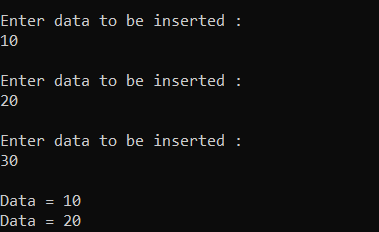Delete at a given position: Delete an element from a specified position in the linked list. Below is the program for the same:

C

 // C program for the above operation#include #include  // Structure of a linked list nodestruct node {    int info;    struct node* next;}; // Pointer to last node in liststruct node* last = NULL; // Function to add a new node// at the end of the listvoid addatlast(){    // Stores number to be inserted    int data;     // Initialize a new node    struct node* temp;    temp = (struct node*)malloc(sizeof(struct node));     // Input data    printf("\nEnter data to be inserted : \n");    scanf("%d", &data);     // If the new node is the    // only node in the list    if (last == NULL) {        temp->info = data;        temp->next = temp;        last = temp;    }     // Else the new node will be    // last node and will contain    // the reference of head node    else {        temp->info = data;        temp->next = last->next;        last->next = temp;        last = temp;    }} // Function to delete an element// at a specified index in the listvoid deleteAtIndex(){    // Stores the index at which    // the element is to be deleted    int pos, i = 1;    struct node *temp, *position;    temp = last->next;     // If list is empty    if (last == NULL)        printf("\nList is empty.\n");     // Else    else {         // Input Data        printf("\nEnter index : ");        scanf("%d", &pos);         // Traverse till the node to        // be deleted is reached        while (i <= pos - 1) {            temp = temp->next;            i++;        }         // After the loop ends, temp        // points at a node just before        // the node to be deleted         // Reassigning links        position = temp->next;        temp->next = position->next;         free(position);    }} // Function to print the listvoid viewList(){    // If list is empty    if (last == NULL)        printf("\nList is empty\n");     // Else print the list    else {        struct node* temp;        temp = last->next;        do {            printf("\nData = %d", temp->info);            temp = temp->next;        } while (temp != last->next);    }} // Driver Codeint main(){    // Initialize the list    addatlast();    addatlast();    addatlast();     // Function Call    deleteAtIndex();     // Print the list    viewList();     return 0;}

Output: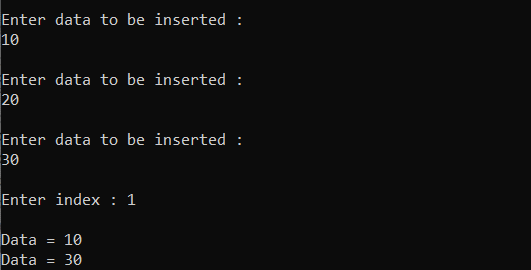My Personal Notes arrow_drop_up